1800-1023-196

+91-120-4616500

# Exceptional Cases Evaluation-II﻿

## Exceptional Cases Evaluation - II

In molecules containing covalent and coordinate bonds, following rules are used for evaluating the oxidation numbers of atoms.

(i) For each covalent bond between dissimilar atoms the less electronegative element is assigned the oxidation number of + 1 while the atom of the more electronegative element is assigned the oxidation number of –1.

(ii) In case of a coordinate-covalent bond between similar or dissimilar atoms but the donor atom is less electronegative than the acceptor atom, an oxidation number of +2 is assigned to the donor atom and an oxidation number of –2 is assigned to the acceptor atom.

Conversely, if the donor atom is more electronegative than the acceptor atom, the contribution of the coordinate bond is neglected. Examples,

(a) Oxidation number of C in HC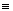N and HN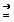C

The evaluation of oxidation number of C cannot be made directly by usual rules since no standard rule exists for oxidation numbers of N and C.

In such cases, evaluation of oxidation number should be made using indirect concept or by the original concepts of chemical bonding.

(b) Oxidation number of carbon in H – NC

The contribution of coordinate bond is neglected since the bond is directed from a more electronegative N atom (donor) to a less electronegative carbon atom (acceptor).

Therefore the oxidation number of N in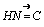remains – 3 as it has three covalent bonds.

1 × (+ 1) + 1 × (– 3) + x = 0

(for H) (for N) (for C)

or 1 + x – 3 = 0 or x = + 2.

(c) Oxidation number of carbon in HC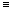N

In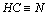, N is more electronegative than carbon, each bond gives an oxidation number of –1 to N. There are three covalent bonds, the oxidation number of N inis taken as – 3

Now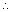+1 + x – 3 = 0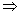x = + 2

## NEET & AIIMS Exam Sample Papers

 AIIMS SAMPLE PAPERS View More NEET SAMPLE PAPERS View More